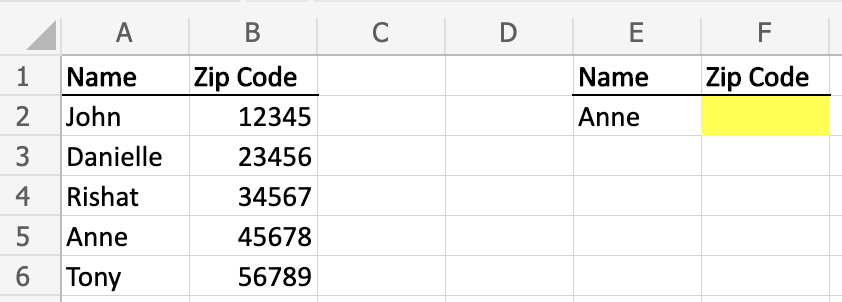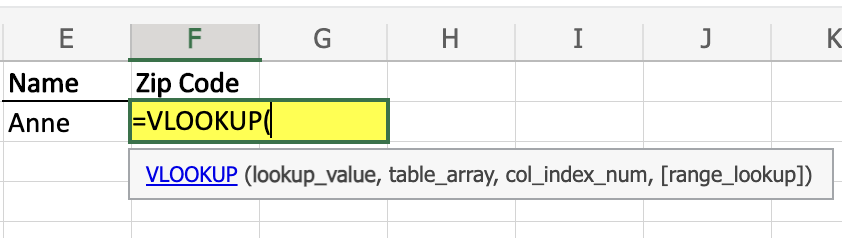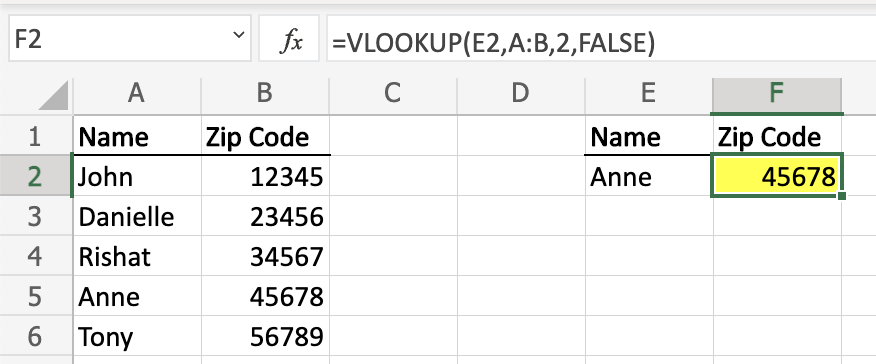#### How to VLOOKUP Zip Codes?

Whether for a school project or a work assignment, chances are you’re going to need to use VLOOKUP at least once in your life. Let’s say you have a long list of names, contact information, and addresses, including zip codes.

You need to pull the zip codes for a targeted subset of contacts on the list, but looking up each contact individually is time consuming. Fortunately, VLOOKUP can help you quickly pull zip codes for any sized list of contacts. Let’s take a look how to VLOOKUP zip codes.

Start by organizing your data into two separate Excel lists. The first list should contain a list of zip codes, and the second one should contain the information you want to return based on the zip code, such as the city, state, or latitude and longitude coordinates.

In this example, you have a list of contacts and need to quickly identify Anne’s zip code from the contact sheet. For this example, we are using a short list for simplicity, but the same concepts can be used if the list is 10 rows long or 10,000 rows long.

Learning materials are available under this link.1. First, we need to identify what we are looking for. In this example, we are using VLOOKUP to identify Anne’s zip code.

2. In order to identify her zip code, we need to understand the VLOOKUP formula.

A VLOOKUP formula looks for a value in the leftmost column of a table, and then returns a value in the same row from a column you specify. It is broken up into four distinct parts (the value, the range you’re looking in, the column that houses the information you want to pull into the cell, and whether or not you need an exact match of what the value is)## Vlookup zip code formula

3. To successfully pull in Anne’s zip code, structure the formula as follows: =VLOOKUP(E2,A:B,2,FALSE)

• E2 – points to Anne, meaning we are looking up Anne in our range
• A:B – highlights the columns where we are locating the lookup value and the value we want to pull into the cell (the zip code)
• 2 – identifies the column housing the information we want to populate in the cell. In this example, the zip code is found in the second column of our highlighted range
• FALSE – means it should only pull information with an exact match (if there were no Anne, or the name was spelled differently, it would not pull a zip code into the cell).To make it easier to identify the information returned based on the zip code, you can use conditional formatting. Conditional formatting allows you to apply a fill color, font color, or other formatting to a cell or range of cells based on a condition.

## How to Look Up Multiple Zip Codes

In addition to looking up a single zip code, the VLOOKUP function can also be used to look up multiple zip codes. This can be done by using the wildcard character (*) in the lookup_value argument.

The wildcard character (*) can be used to represent any number of characters. For example, the following formula would look up all of the zip codes that start with the number “123” in the range A2:A10:

`=VLOOKUP("*123*, A2:A10, 2, FALSE)`

This formula would return the city in the corresponding cell in the range B2:B10 for all of the zip codes that start with the number “123”.

Image of VLOOKUP function to look up multiple zip codes

The VLOOKUP function can also be used to look up multiple zip codes by using the MATCH function. The MATCH function is used to find the position of a value in a range.

The following formula would find the position of the first occurrence of the zip code “12345” in the range A2:A10:

`=MATCH("12345", A2:A10, 0)`

The MATCH function returns an integer value that represents the position of the value in the range. This value can then be used as the col_index_num argument of the VLOOKUP function to find the corresponding value in another range.

For example, the following formula would look up all of the zip codes that are located in the state of California in the range A2:A10:

`=VLOOKUP(MATCH("CA", A2:A10, 0), A2:A10, 2, FALSE)`

This formula would return the city in the corresponding cell in the range B2:B10 for all of the zip codes that are located in the state of California.